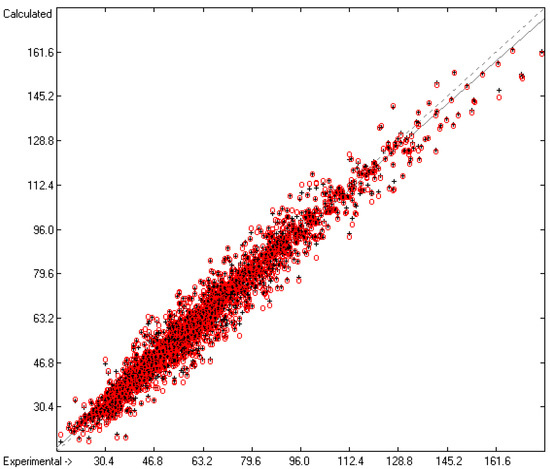Previous Article in Journal
Recent Advances in Conotoxin Classification by Using Machine Learning Methods
Open AccessArticle

# Calculation of Five Thermodynamic Molecular Descriptors by Means of a General Computer Algorithm Based on the Group-Additivity Method: Standard Enthalpies of Vaporization, Sublimation and Solvation, and Entropy of Fusion of Ordinary Organic Molecules and Total Phase-Change Entropy of Liquid Crystals

byRudolf Naef 1,*andWilliam E. Acree 2
1
Department of Chemistry, University of Basel, Basel 4003, Switzerland
2
Department of Chemistry, University of North Texas, Denton, TX 76203, USA
*
Author to whom correspondence should be addressed.
Molecules 2017, 22(7), 1059; https://doi.org/10.3390/molecules22071059
Received: 22 May 2017 / Revised: 22 June 2017 / Accepted: 22 June 2017 / Published: 25 June 2017
The calculation of the standard enthalpies of vaporization, sublimation and solvation of organic molecules is presented using a common computer algorithm on the basis of a group-additivity method. The same algorithm is also shown to enable the calculation of their entropy of fusion as well as the total phase-change entropy of liquid crystals. The present method is based on the complete breakdown of the molecules into their constituting atoms and their immediate neighbourhood; the respective calculations of the contribution of the atomic groups by means of the Gauss-Seidel fitting method is based on experimental data collected from literature. The feasibility of the calculations for each of the mentioned descriptors was verified by means of a 10-fold cross-validation procedure proving the good to high quality of the predicted values for the three mentioned enthalpies and for the entropy of fusion, whereas the predictive quality for the total phase-change entropy of liquid crystals was poor. The goodness of fit (Q2) and the standard deviation (σ) of the cross-validation calculations for the five descriptors was as follows: 0.9641 and 4.56 kJ/mol (N = 3386 test molecules) for the enthalpy of vaporization, 0.8657 and 11.39 kJ/mol (N = 1791) for the enthalpy of sublimation, 0.9546 and 4.34 kJ/mol (N = 373) for the enthalpy of solvation, 0.8727 and 17.93 J/mol/K (N = 2637) for the entropy of fusion and 0.5804 and 32.79 J/mol/K (N = 2643) for the total phase-change entropy of liquid crystals. The large discrepancy between the results of the two closely related entropies is discussed in detail. Molecules for which both the standard enthalpies of vaporization and sublimation were calculable, enabled the estimation of their standard enthalpy of fusion by simple subtraction of the former from the latter enthalpy. For 990 of them the experimental enthalpy-of-fusion values are also known, allowing their comparison with predictions, yielding a correlation coefficient R2 of 0.6066. View Full-Text
Show FiguresFigure 1

MDPI and ACS Style

Naef, R.; Acree, W.E. Calculation of Five Thermodynamic Molecular Descriptors by Means of a General Computer Algorithm Based on the Group-Additivity Method: Standard Enthalpies of Vaporization, Sublimation and Solvation, and Entropy of Fusion of Ordinary Organic Molecules and Total Phase-Change Entropy of Liquid Crystals. Molecules 2017, 22, 1059.